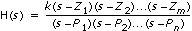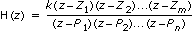# Zero-Pole-Gain

Creates a single-input single-output (SISO) zero-pole-gain model of a system using the Zeros, Poles, Gain, and Sampling Time (s). This step also can produce a zero-pole-gain model in which the data is specified in symbolic form.

ParameterDescription
NameSpecifies the name of the model you create.
InputsDisplays the number of inputs in the system model.
OutputsDisplays the number of outputs in the system model.
Model TypeSpecifies the type of model you create. Contains the following options:
• Continuous—Specifies that the model you create is in the continuous time domain.
• Discrete—Specifies that the model you create is in the discrete time domain. When you place a checkmark in the Discrete checkbox, the Sampling Time (s) parameter appears.
• Sampling Time (s)—Defines the sampling time used in the discrete model. The default is 1. By using the coefficients of the continuous model and setting the Sampling Time (s) of the system to a value greater than zero, you do not create the discrete-time equivalent of the system. If you create a continuous model and want to convert the model to a discrete model, you must use the Discretize Model step.

If you already know the coefficients of a discrete transfer function model, you can create the model using this step. Enter the coefficients for the discrete model and set the Sampling Time (s) of the system to a value greater than zero.
Symbolic CoefficientsSpecifies that you can use variables to define the model. Define the variable name and the value that the variable represents in the left and right columns, respectively, that appear in the Symbolic coefficients section.
• Number of Variables—Specifies the maximum number of variables used. The maximum number of variables you can specify is nine.
• Variables—Lists the coefficient variables and values. The number of variables you specify in the Number of Variables control corresponds to the number of rows in the Variables array.
Resulting ModelDisplays the mathematical expression that defines the model you create.
Gain Defines the scalar gain of the model. The default is 1.
ZerosDefines the array of zeros of the model. The zeros can be real or complex. If they are complex, they must be in complex conjugate pairs.
PolesDefines the array of poles of the model. The poles can be real or complex. If they are complex, they must be in complex conjugate pairs.
Generate Random ModelGenerates a random model.

## Zero-Pole-Gain Details

The zero-pole-gain model is defined by the following equations:

 ContinuousDiscrete